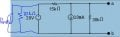# Voltage source and Current source in parallel

#### Sagan820

Joined Jul 10, 2016
19Hello,
So I was asked to find the Norton Equivalent of the circuit above. The solution stated that the 8mA current and the 20kOhms resistor have no effect on the circuit with respect to terminals a,b and, hence, can be removed. From what I can understand, an ideal current source will take on any value of voltage and vice versa; so the 8mA current source will act the same as a 30v voltage source in place. What I don't understand is why the current source and the resistor have no effects on the circuit and would it be the same if I removed the 30v voltage source instead of the current source? Please help!

Thank you!

#### DGElder

Joined Apr 3, 2016
351
an ideal current source will take on any value of voltage and vice versa

And vice versa? What do you mean?

I don't understand is why the current source and the resistor have no effects on the circuit and would it be the same if I removed the 30v voltage source instead of the current source?

The only connection of that pair to the circuit is at the two nodes of an ideal voltage source that locks those nodes at a 30V differential - the current source can't change that voltage. The terminals of the 30V voltage source, along with the 10ma current source, drive all the currents and voltages in the network to the right of the 30V source. There is nothing to the left of the 30V source that could possibly influence the voltage at a,b. If you remove the 30V source then the 30V lock at its terminal nodes is removed and the voltage would change.

Perhaps your confusion comes from not realizing that a current source can also act as a current sink and that is the case here. You can see that because the voltage across the CS (due to the ideal voltage source) is reversed from the current direction such that it is absorbing power, not delivering power. In this situation it is indistinguishable from a 3.75K resistor ( 30V / 8ma ). In parallel with the 20K you basically have an equivalent 3.158K resistor in parallel with the 30V source. You can see that any equivalent resistance value will have no effect on the the network to the right of the ideal voltage source.

Last edited:
•Sagan820

#### WBahn

Joined Mar 31, 2012
25,904
An ideal voltage source isolates anything that is in parallel with it from each other. The reason is that it will force a fixed voltage across them all and will produce or absorb whatever current each of them needs.

No it would not be the same if you removed the current source instead. Let's see if we can make this fact really obvious. What if the current source was 0 mA instead of 8 mA? What would happen if you then removed the 30 V source? But what if the 8 mA source wasn't there to begin with? Wouldn't you expect that 30 V source to have an effect on the voltage seen at the terminals? But these are the same situation -- a current source set to 0 mA is an open circuit and can be removed. Similarly, what if the current source was 1000 A instead of 8 mA? If you remove the 30 V source, then most of that current has to flow though the 20 Ω resistor resulting in a huge voltage where, presently, the 30 V source clamps it to 30 V.

An ideal current source isolates anything that is in series with it from each other. The reason is that it will force a fixed current through them all and will produce whatever voltage, positive or negative, that is needed to do this.

•Sagan820

#### Sagan820

Joined Jul 10, 2016
19
What I am confused
an ideal current source will take on any value of voltage and vice versa

And vice versa? What do you mean?

I don't understand is why the current source and the resistor have no effects on the circuit and would it be the same if I removed the 30v voltage source instead of the current source?

The only connection of that pair to the circuit is at the two nodes of an ideal voltage source that locks those nodes at a 30V differential - the current source can't change that voltage. The terminals of the 30V voltage source, along with the 10ma current source, drive all the currents and voltages in the network to the right of the 30V source. There is nothing to the left of the 30V source that could possibly influence the voltage at a,b. If you remove the 30V source then the 30V lock at its terminal nodes is removed and the voltage would change.

Perhaps your confusion comes from not realizing that a current source can also act as a current sink and that is the case here. You can see that because the voltage across the CS (due to the ideal voltage source) is reversed from the current direction such that it is absorbing power, not delivering power. In this situation it is indistinguishable from a 3.75K resistor ( 30V / 8ma ). In parallel with the 20K you basically have an equivalent 3.158K resistor in parallel with the 30V source. You can see that any equivalent resistance value will have no effect on the the network to the right of the ideal voltage source.
This makes complete sense! I got myself confused when I tried to use the superposition method to see how the CS affect the circuit and I made a mistake by replace the voltage source with an open circuit instead of a short circuit, which would affect how the current flow through the 10k resistor. Thank you!